ISEE Lower Level Quantitative : How to compare fractions

Example Questions

← Previous 1 3 4 5 6

Example Question #17 : How To Order Fractions From Least To Greatest Or From Greatest To Least

Select the symbol to correctly fill in the blank below.__________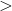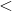Explanation:

To compare fractions, we need to first make common denominators.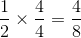Now that we have common denominators, we can compare numerators. The fraction with the bigger numerator has the greater value.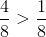Example Question #321 : Fractions

Select the symbol to correctly fill in the blank below.__________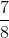Explanation:

To compare fractions, we need to first make common denominators.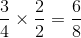Now that we have common denominators, we can compare numerators. The fraction with the bigger numerator has the greater value.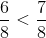Example Question #21 : Number & Operations: Fractions

Select the symbol to correctly fill in the blank below.__________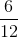Explanation:

To compare fractions, we need to first make common denominators.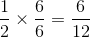Now that we have common denominators, we can compare numerators. The fraction with the bigger numerator has the greater value.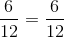Example Question #1691 : Numbers And Operations

Select the symbol to correctly fill in the blank below.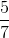__________Explanation:

To compare fractions, we need to first make common denominators.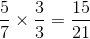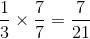Now that we have common denominators, we can compare numerators. The fraction with the bigger numerator has the greater value.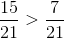Example Question #2 : How To Compare Fractions

Select the symbol to correctly fill in the blank below.__________Explanation:

To compare fractions, we need to first make common denominators.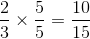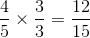Now that we have common denominators, we can compare numerators. The fraction with the bigger numerator has the greater value.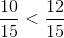Example Question #3 : How To Compare Fractions

Select the symbol to correctly fill in the blank below.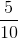__________Explanation:

To compare fractions, we need to first make common denominators.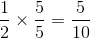Now that we have common denominators, we can compare numerators. The fraction with the bigger numerator has the greater value.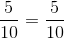Example Question #1 : How To Compare Fractions

Select the symbol to correctly fill in the blank below.__________Explanation:

To compare fractions, we need to first make common denominators.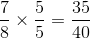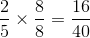Now that we have common denominators, we can compare numerators. The fraction with the bigger numerator has the greater value.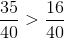Example Question #56 : Fractions

Select the symbol to correctly fill in the blank below.__________Explanation:

To compare fractions, we need to first make common denominators.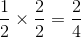Now that we have common denominators, we can compare numerators. The fraction with the bigger numerator has the greater value.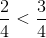Example Question #32 : Number & Operations: Fractions

Select the symbol to correctly fill in the blank below.__________Explanation:

To compare fractions, we need to first make common denominators.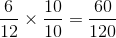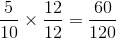Now that we have common denominators, we can compare numerators. The fraction with the bigger numerator has the greater value.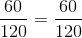Example Question #3 : Compare Two Fractions With Different Numerators And Different Denominators: Ccss.Math.Content.4.Nf.A.2

Select the symbol to correctly fill in the blank below.__________Explanation:

To compare fractions, we need to first make common denominators.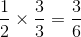Now that we have common denominators, we can compare numerators. The fraction with the bigger numerator has the greater value.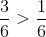← Previous 1 3 4 5 6